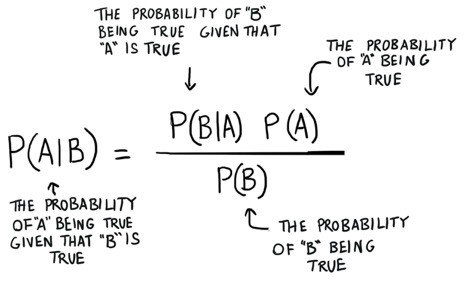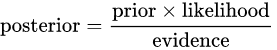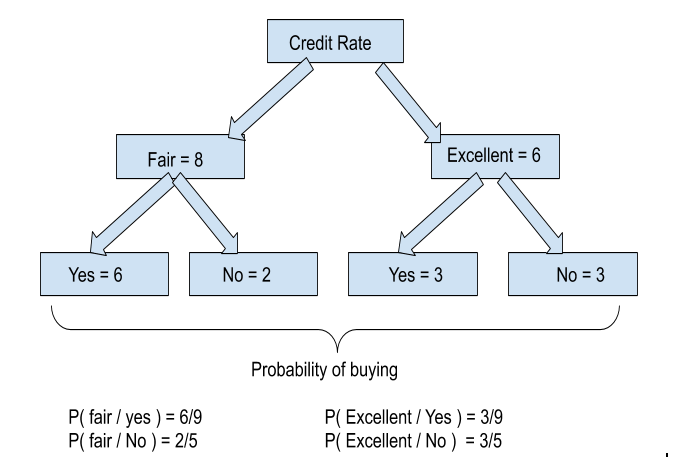# NAIVE BAYES ALGORITHM FROM SCRATCHIn our previous Machine Learning From Scratch series, we have covered Linear Regression and Logistic Regression. Today, we will be covering all details about Naive Bayes Algorithm from scratch.

Naive Bayes is a classification algorithm based on the “Bayes Theorem”. So let’s get introduced to the Bayes Theorem first.

Bayes Theorem is used to find the probability of an event occurring given the probability of another event that has already occurred. Here B is the evidence and A is the hypothesis. Here P(A) is known as prior, P(A/B) is posterior, and P(B/A) is the likelihood.#### NAIVE BAYES ALGORITHM

The name Naive is used because the presence of one independent feature doesn’t affect (influence or change the value of) other features. The most important assumption that Naive Bayes makes is that all the features are independent of each other. Being less prone to overfitting, Naive Bayes algorithm works on Bayes theorem to predict unknown data sets.

##### EXAMPLE:

We are given a table that contains a dataset about age, income, student, credit-rating, buying a computer, and their respective features. From the above dataset, we need to find whether a youth student with medium income having a fair credit rating buys a computer or not.

I.e. B = (Youth, Medium, Yes, Fair)

In the above dataset, we can apply the Bayesian theorem.

Where,

• A = ( Yes / No ) under buying computer
• B = ( Youth, Medium, Student, Fair)

So, P(A/B) means the probability of buying a computer given that conditions are “Youth age”, “Medium Income”, “Student”, and “fair credit-rating”.

##### ASSUMPTION:

Before starting, we assume that all the given features are independent of each other.

##### Step 2: Calculate probabilities under Credit-rating buying a computer from the above datasetLet’s understand how we calculated the above probabilities. From the table we can see that there are 8 fair credit ratings among which 6 buy computers and 2 don’t buy. Similarly, 6 have excellent credit ratings among which 3 buy computers and 3 don’t. As a whole 9 (6+3)  buy computers and 5 (2+5) don’t.

P(fair / Yes) means the probability of credit rating being fair when someone buys a computer. Hence, P(fair / Yes) = P( fair buying computer) / P ( total number of buying computer) i.e. 6/9.

##### Step 3: Calculate probabilities under Student buying a computer from the above dataset##### Step 4: Calculate probabilities under Income level  buying a computer from the above dataset

P( High / Yes ) = 2/9       P( Mid / Yes ) = 4/9               P( Low / Yes ) = 3/9

P( High / No ) = 2/5        P( Mid / No ) = 2/5                 P( Low / No ) =  1/5

##### Step 5: Calculate probabilities under age level  buying a computer from the above dataset

P( Youth / Yes ) = 2/9       P( Mid / Yes ) = 4/9               P( Senior / Yes ) = 3/9

P( Youth / No ) = 3/5        P( Mid / No ) = 0                    P( Senior / No ) =  ⅖

##### CALCULATION

We have,

B = ( Youth, Medium, Student, Fair)

P(Yes) * P(B / Yes )  = P(Yes) * P( Youth / Yes ) * P( Mid / Yes) * P( S Yes / Yes) * P( Fair / Yes)

= 9/14 * 2/9 * 4/9 * 6/9 * 6/9

= 0.02821

P(No) * P(B / No ) = P(No) * P( Youth / No ) * P( Mid / No) * P( S Yes / No) * P( Fair / No)

= 5/14 * 3/5 * 2/5 * 1/5 * 2/5

= 0.0068

P( B ) = P (Youth) * P( Mid) * P(Student Yes) * P( Fair)

=  5/14 * 6/14 * 7/14 * 8/14

= 0.04373

Hence,

P(Yes / B) = P(Yes) * P(B / Yes )  / P(B)

= 0.02821 / 0.04373

= 0.645

P(No / B) = P(No) * P(B / No )  / P(B)

= 0.0068 / 0.04373

= 0.155

Here,  P(Yes / B) is greater than  P(No / B) i.e posterior Yes is greater than posterior No. So the class B ( Youth, Mid, yes, fair) buys a computer.

#### NAIVE BAYES FROM SCRATCH

###### Classify whether a given person is a male or a female based on the measured features. The features include height, weight, and foot size.

Now, defining a dataframe which consists if above provided data.

Creating another data frame containing the feature value of height as 6 feet, weight as 130 lbs and foot size as 8 inches. using Naive Bayes from scratch we are trying to find whether the gender is male or female.

Calculating the total number of males and females and their probabilities i.e priors:

Calculating mean and variance of male and female of the feature height, weight and foot size.

##### FORMULA

• posterior (male) = P(male)*P(height|male)*P(weight|male)*P(foot size|male) / evidence
• posterior (female) = P(female)*P(height|female)*P(weight|female)*P(foot size|female) / evidence
• Evidence = P(male)*P(height|male)*P(weight|male)*P(foot size|male) + P(female) * P(height|female) * P(weight|female)*P(foot size|female)

The evidence may be ignored since it is a positive constant. (Normal distributions are always positive.)

##### CALCULATION

###### Calculation of P(height | Male )

• mean of  the height of male = 5.855
• variance ( Square of S.D.)  of the height of a male is square of 3.5033e-02
• and x i.e. given height is 6 feet
• Substituting the values in the above equation we get  P(height | Male ) = 1.5789

Similarly,

• P(weight|male) = 5.9881e-06
• P(foot size|male) = 1.3112e-3
• P(height|female) = 2.2346e-1
• P(weight|female) = 1.6789e-2
• P(foot size|female) = 2.8669e-1

Posterior (male)*evidence = P(male)*P(height|male)*P(weight|male)*P(foot size|male) = 6.1984e-09

Posterior (female)*evidence = P(female)*P(height|female)*P(weight|female)*P(foot size|female)= 5.3778e-04

##### CONCLUSION

Since Posterior (female)*evidence > Posterior (male)*evidence, the sample is female.

#### NAIVE BAYES USING SCIKIT-LEARN

Though we have very small dataset, we are dividing the dataset into train and test do that it can be used in other model prediction. We are importing gnb() from sklearn and we are training the model with out dataset.

Now, our model is ready. Let’s use this model to predict on new data.

##### LAPLACE SMOOTHING

Laplace smoothing, often affectionately referred to as “add-one smoothing” by data nerds, comes to the rescue when Naive Bayes finds itself in a bit of a statistical pickle. Let’s break down how this technique saves the day for the nerdy world of Naive Bayes.

###### The Problem: Zero Probabilities in Naive Bayes

In Naive Bayes classification, we’re trying to predict the probability of a data point belonging to a particular class based on its features. Naive Bayes assumes that the features are independent, which simplifies the calculations. However, this simplification can lead to a significant problem, especially in cases where we have limited data.

Imagine you’re dealing with text data and trying to classify documents into categories based on the words they contain. The trouble arises when a particular word (a feature) doesn’t appear in documents of a certain category during training. This means that when Naive Bayes calculates the probability of that word occurring in that category, it’s a big fat zero!

###### The Solution: Laplace Smoothing to the Rescue

This is where Laplace smoothing steps in like a trusty sidekick. Laplace smoothing is all about adding some extra oomph to those zero probabilities. Here’s how it works:

1. Counting Feature Occurrences: During the training phase, we meticulously count how often each feature (in our case, words) appears in each category. This gives us an idea of the probability of seeing a particular feature in a given category.

2. Smoothing Magic:  Now, instead of letting any count hit rock bottom at zero, Laplace smoothing steps in. For each feature-category pair, we add one to the count. This ensures that no count is zero, and every feature has a tiny chance of appearing in any category.

3. Balancing Act: We adjust the feature probabilities and overall probabilities of each category. This adjustment ensures that probabilities remain consistent and that one category doesn’t become too dominant.

###### Why It’s Nerdy and Awesome

Laplace smoothing is nerdy in the best way possible because it’s all about bridging the gap between theory and reality. In theory, we’d love to work with precise probabilities, but in the real world, data can be sparse, and zeros can be problematic.

By adding just one to every count, Laplace smoothing allows Naive Bayes to handle data imperfections and gracefully avoid those dreaded zero probabilities. This makes our Naive Bayes classifier more robust, less prone to overfitting, and ready to tackle classification problems with confidence.

So, next time you see those “add-one” adjustments in your Naive Bayes calculations, you can appreciate how nerdy but essential Laplace smoothing makes your classifier a true data superhero.

##### CONCLUSION

Naive Bayes, in conjunction with Laplace smoothing, is a valuable and straightforward classification algorithm, particularly adept at managing categorical and discrete data. Its computational efficiency and resilience when dealing with sparse datasets and unobserved feature-value combinations make it especially suitable for text classification tasks, including spam detection, sentiment analysis, and document categorization. Although its feature independence assumption may not always hold in intricate datasets, Naive Bayes and Laplace smoothing serve as a dependable baseline model and a resource-efficient option, particularly when dealing with limited computational resources or real-time processing requirements. However, it’s essential to carefully assess the characteristics of your data and the specific problem domain to determine whether Naive Bayes is the appropriate choice, as more complex algorithms may outperform it in situations with pronounced feature interdependencies.🚀 I'm Diwas Pandey, a Computer Engineer with an unyielding passion for Artificial Intelligence, currently pursuing a Master's in Computer Science at Washington State University, USA. As a dedicated blogger at AIHUBPROJECTS.COM, I share insights into the cutting-edge developments in AI, and as a Freelancer, I leverage my technical expertise to craft innovative solutions. Join me in bridging the gap between technology and healthcare as we shape a brighter future together! 🌍🤖🔬

View all posts by Diwas →

### 24 Comments on “NAIVE BAYES ALGORITHM FROM SCRATCH”

1.vreyro linomit says:

Merely wanna remark that you have a very decent web site, I love the design it really stands out.

2.Lav@ says:

Look forward to exploring your web page for a second time.

3.Demske says:

I do agree with all the ideas you have presented in your post.

4.Philip says:

Hello! I just wish to give a huge thumbs up for the good info you have right here on this post. I will be coming back to your weblog for more soon.

5.Newmann says:

Does your website have a contact page? I’m having problems locating it but, I’d like to send you an email. I’ve got some creative ideas for your blog you might be interested in hearing. Either way, great blog and I look forward to seeing it develop over time.

6.Flick says:

Wow! This can be one particular of the most beneficial blogs We’ve ever arrive across on this subject. Basically Excellent. I am also a specialist in this topic therefore I can understand your effort.

7.Halpainy says:

Thank you for sharing excellent informations.

8.Lorino says:

Woah! I’m really enjoying Exceptional Blog!

9.Litz says:

Nice blog here!

10. Pingback: URL
11.Ruden says:

Informative Site Hello guys here are some links that contains information that you may find useful yourselves. Its Worth Checking out.

12.Donnie Kasperek says:

Keep working ,splendid job!

13.Estell Sessa says:

It’s really a cool and useful piece of info. I’m glad that you shared this helpful info with us. Please keep us up to date like this. Thanks for sharing.

14.Leo Dornbos says:

This really answered my downside, thank you!

15.Paul N. says:

In step 3, you have 7/9 as P(S Yes/Yes). I think this is wrong and should be 6/9. Unfortunately this means all the results you calculate at the end of the example need to be fixed.

Please let me know if you agree or if I am missing something.

1.Diwas Pandey says:

yeah, that was a typing mistake and now it’s corrected. The calculation part is correct. Thank you for pointing it out.

16.Sindy Closs says:

The condition associated with the person seeking medical care is theimportant factor in your choice associated with a long-term care service.

17.web hosting company says:

18.hosting services says: